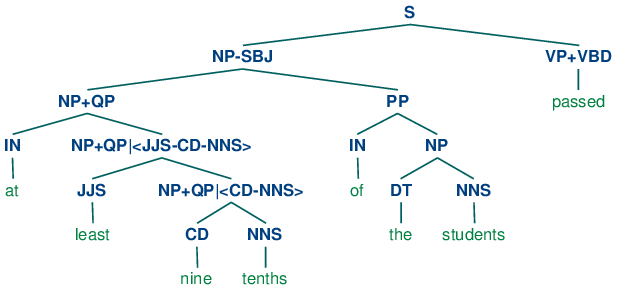# 1   Chunking vs Parsing

You might be asking what's new about phrasal constituents, since they are very similar to the chunks presented in 7.. To answer this, let's return to the challenge of making explicit how a sentence says "who did what to whom". Let's just focus for a while on the "who" part of this story. As a first approximation, we'll say that this amounts to telling what the grammatical subject of a sentence is. Can we do this using chunks? Based on the techniques described in 7., a chunking analysis of (6) will look as follows:

```(S
(NP the/DT little/JJ bear/NN)
saw/VBD
(NP the/DT fine/JJ fat/JJ trout/NN)
in/IN
(NP the/DT brook/NN))
```

So we might adopt the heuristic that the subject of a sentence is the NP chunk that immediately precedes the tensed verb: this would correctly yield (NP the/DT little/JJ bear/NN) as subject. Unfortunately, this simple rule very quickly fails, as shown by a more complex example.

 ```>>> from nltk.corpus import conll2000 >>> print conll2000.chunked_sents() (S Chancellor/NNP (PP of/IN) (NP the/DT Exchequer/NNP) (NP Nigel/NNP Lawson/NNP) (NP 's/POS restated/VBN commitment/NN) (PP to/TO) (NP a/DT firm/NN monetary/JJ policy/NN) (VP has/VBZ helped/VBN to/TO prevent/VB) (NP a/DT freefall/NN) (PP in/IN) (NP sterling/NN) (PP over/IN) (NP the/DT past/JJ week/NN) ./.)```

What's doing the "preventing" in this example is not the firm monetary policy, but rather the restated commitment to such a policy. We can also see from this example that a different simple rule, namely treating the initial NP chunk as the subject, also fails, since this would give us the (NP the/DT Exchequer/NNP). By contrast, a complete phrase structure analysis of the sentence would group together all the pre-verbal NP chunks into a single NP constituent:

 (1)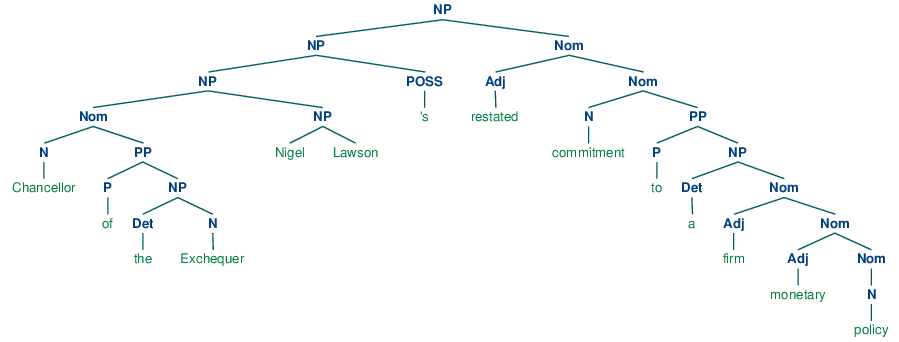We still have a little work to determine which part of this complex NP corresponds to the "who", but nevertheless, this is much more tractable than answering the same question from a flat sequence of chunks.

"Subject" and "direct object" are examples of grammatical functions. Although they are not captured directly in a phrase structure grammar, they can be defined in terms of tree configurations. In (2), the subject of S is the NP immediately dominated by S while the direct object of V is the NP directly dominated by VP.

 (2)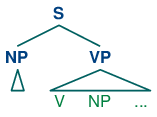# 2   Chart Parsing

Chart Parsing is a parsing algorithm that uses dynamic programming, a technique described in 4.7. It extends the method of well-formed substring tables from 4.

## 2.1   Active Charts

The content of a WFST can be represented in a directed acyclic graph, as shown in (3a) for the initialized WFST and (3b) for the completed WFST.

(3)

 a.b.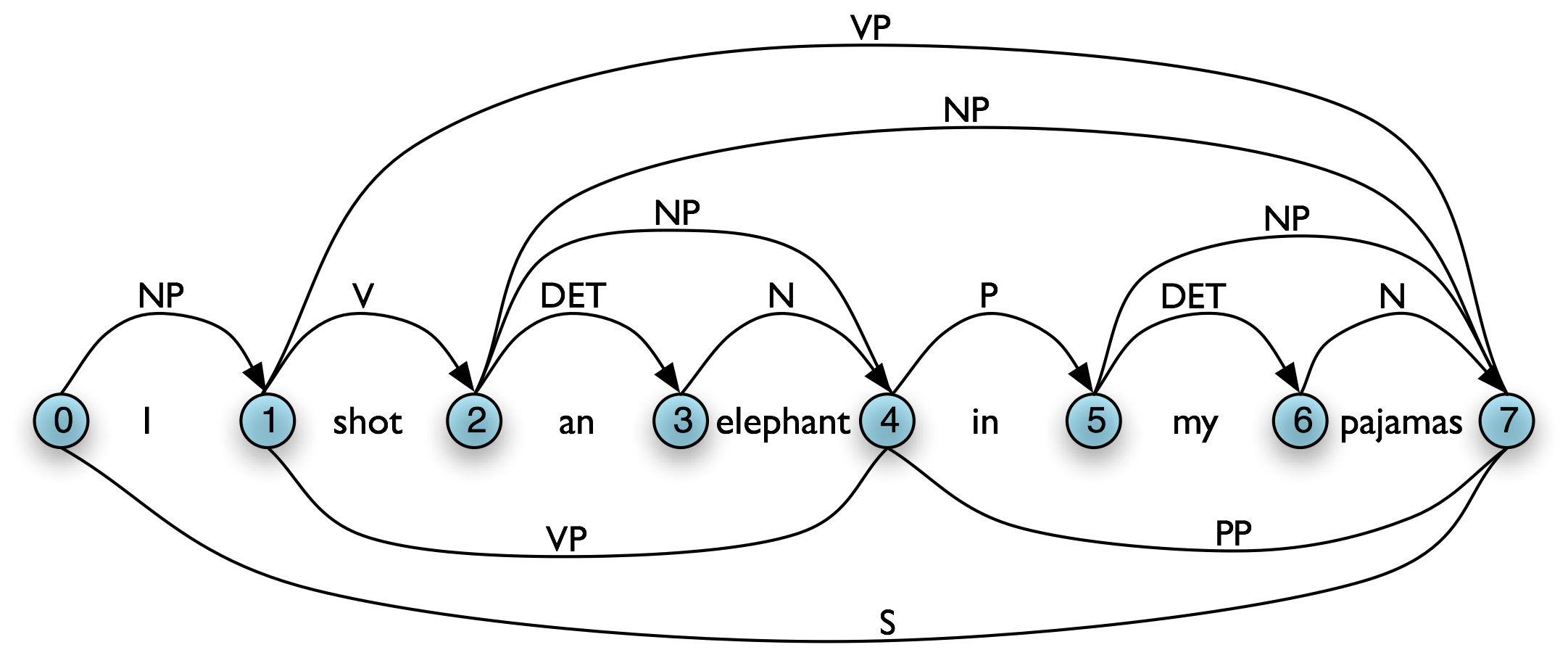In general, a chart parser hypothesizes constituents (i.e. adds edges) based on the grammar, the tokens, and the constituents already found. Any constituent that is compatible with the current knowledge can be hypothesized; even though many of these hypothetical constituents will never be used in the final result. A WFST just records these hypotheses.

All of the edges that we've seen so far represent complete constituents. However, it is helpful to record incomplete constituents, to document the work already done by the parser. For example, when a top-down parser processes VPV NP PP, it may find V and NP but not the PP. This work can be reused when processing VPV NP. Thus, we will record the hypothesis that "the V constituent likes is the beginning of a VP."

We can do this by adding a dot to the edge's right hand side. Material to the left of the dot records what has been found so far; material to the right of the dot specifies what still needs to be found in order to complete the constituent. For example, the edge in (4) records the hypothesis that "a VP starts with the V likes, but still needs an NP to become complete":

 (4)## 2.2   Types of Edge

Let's take stock. An edge [VP → • V NP PP, (i, i)] records the hypothesis that a VP begins at location i, and that we anticipate finding a sequence V NP PP starting here. This is known as a self-loop edge; see (5a). An edge [VPVNP PP, (i, j)] records the fact that we have discovered a V spanning (i, j), and hypothesize a following NP PP sequence to complete a VP beginning at i. This is known as an incomplete edge; see (5b). An edge [VPV NP PP • , (i, k)] records the discovery that a VP consisting of the sequence V NP PP has been discovered for the span (i, j). This is known as a complete edge; see (5c). If a complete edge spans the entire sentence, and has the grammar's start symbol as its left-hand side, then the edge is called a parse edge, and it encodes one or more parse trees for the sentence; see (5c).

(5)

 a.b.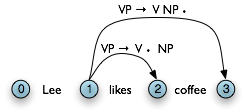c.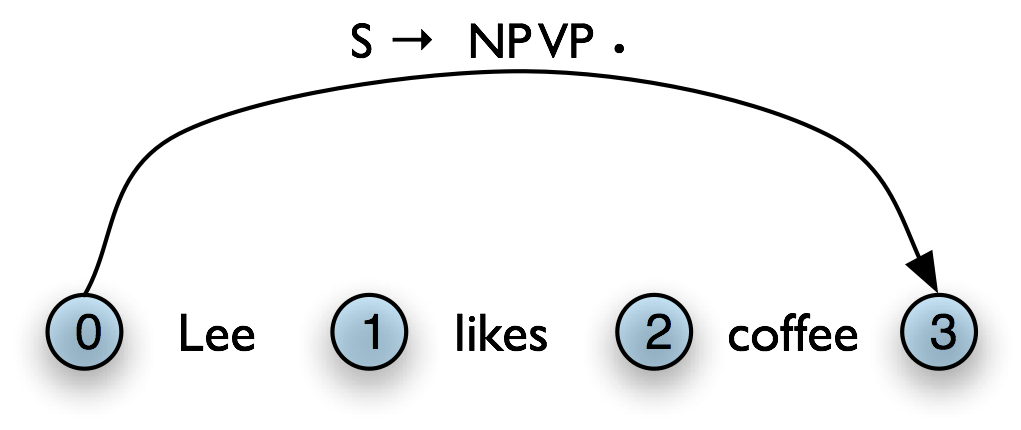## 2.3   The Chart Parser

To parse a sentence, a chart parser first creates an empty chart spanning the sentence. It then finds edges that are licensed by its knowledge about the sentence, and adds them to the chart one at a time until one or more parse edges are found. The edges that it adds can be licensed in one of three ways:

1. The input can license an edge: each word wi in the input licenses the complete edge [wi → •, (i, i+1)].
2. The grammar can license an edge: each grammar production A → α licenses the self-loop edge [A → • α, (i, i)] for every i, 0 ≤ i < n.
3. The current chart contents can license an edge: a suitable pair of existing edges triggers the addition of a new edge.

## 2.4   The Fundamental Rule

One rule is particularly important, since it is used by every chart parser: the Fundamental Rule. This rule is used to combine an incomplete edge that's expecting a nonterminal B with a following, complete edge whose left hand side is B. The rule is defined and illustrated in (6). We will use α, β, and γ to denote (possibly empty) sequences of terminals or non-terminals.

In the new edge, the dot has moved one place to the right. Its span is the combined span of the original edges. Note that in adding this new edge we do not remove the other two, because they might be used again.

## 2.5   Bottom-Up Parsing

As we saw with the shift-reduce parser in 4, bottom-up parsing starts from the input string, and tries to find sequences of words and phrases that correspond to the right hand side of a grammar production. The parser then replaces these with the left-hand side of the production, until the whole sentence is reduced to an S. Bottom-up chart parsing is an extension of this approach in which hypotheses about structure are recorded as edges on a chart. In terms of our earlier terminology, bottom-up chart parsing can be seen as a parsing strategy; in other words, bottom-up is a particular choice of heuristics for adding new edges to a chart.

The general procedure for chart parsing is inductive: we start with a base case, and then show how we can move from a given state of the chart to a new state. Since we are working bottom-up, the base case for our induction will be determined by the words in the input string, so we add new edges for each word. Now, for the induction step, suppose the chart contains an edge labeled with constituent A. Since we are working bottom-up, we want to build constituents that can have an A as a child. In other words, we are going to look for productions of the form BA β and use these to label new edges.

Let's look at the procedure a bit more formally. To create a bottom-up chart parser, we add to the Fundamental Rule two new rules: the Bottom-Up Initialization Rule; and the Bottom-Up Predict Rule. The Bottom-Up Initialization Rule says to add all edges licensed by the input.

Next, suppose the chart contains a complete edge e whose left hand category is A. Then the Bottom-Up Predict Rule requires the parser to add a self-loop edge at the left boundary of e for each grammar production whose right hand side begins with category A.

The next step is to use the Fundamental Rule to add edges like [NP → Lee • , (0, 1)], where we have "moved the dot" one position to the right. After this, we will now be able to add new self-loop edges such as [S → • NP VP, (0, 0)] and [VP → • VP NP, (1, 1)], and use these to build more complete edges.

Note

Your Turn: NLTK provides a useful interactive tool for visualizing the operation of a chart parser: nltk.app.chartparser(). The tool comes with a pre-defined input string and grammar, but both of these can be readily modified with options inside the Edit menu.

## 2.6   Top-Down Parsing

Top-down chart parsing works in a similar way to the recursive descent parser, in that it starts off with the top-level goal of finding an S. This goal is broken down into the subgoals of trying to find constituents such as NP and VP predicted by the grammar. To create a top-down chart parser, we use the Fundamental Rule as before plus three other rules: the Top-Down Initialization Rule, the Top-Down Expand Rule, and the Top-Down Match Rule. The Top-Down Initialization Rule in (10) captures the fact that the root of any parse must be the start symbol S.

In our running example, we are predicting that we will be able to find an NP and a VP starting at 0, but have not yet satisfied these subgoals. In order to find an NP we need to invoke a production that has NP on its left hand side. This work is done by the Top-Down Expand Rule (11). This tells us that if our chart contains an incomplete edge whose dot is followed by a nonterminal B, then the parser should add any self-loop edges licensed by the grammar whose left-hand side is B.

The Top-Down Match rule allows the predictions of the grammar to be matched against the input string. Thus, if the chart contains an incomplete edge whose dot is followed by a terminal w, then the parser should add an edge if the terminal corresponds to the current input symbol.

Here we see our example chart after applying the Top-Down Match rule. After this, we can apply the fundamental rule to add the edge [NP → Lee • , (0, 1)].

Using these four rules, we can parse a sentence top-down as shown in (13).

## 2.7   The Earley Algorithm

The Earley algorithm (Earley, 1970) is a parsing strategy that resembles the Top-Down Strategy, but deals more efficiently with matching against the input string. 2.1 shows the correspondence between the parsing rules introduced above and the rules used by the Earley algorithm.

Table 2.1:

Terminology for rules in the Earley algorithm

Top-Down/Bottom-Up Earley
Top-Down Initialization Rule Top-Down Expand Rule Predictor Rule
Top-Down/Bottom-Up Match Rule Scanner Rule
Fundamental Rule Completer Rule

## 2.8   Chart Parsing in NLTK

NLTK defines a simple yet flexible chart parser, ChartParser. A new chart parser is constructed from a grammar and a strategy. The strategy is applied until no new edges are added to the chart. NLTK defines two ready-made strategies: TD_STRATEGY, a basic top-down strategy; and BU_STRATEGY, a basic bottom-up strategy. When constructing a chart parser, you can use either of these strategies, or create your own. We've already seen how to define a chart parser in 1. This time we'll specify a strategy and turn on tracing:

 ```>>> sent = ['I', 'shot', 'an', 'elephant', 'in', 'my', 'pajamas'] >>> parser = nltk.ChartParser(groucho_grammar, nltk.parse.BU_STRATEGY) >>> trees = parser.nbest_parse(sent, trace=2)```

The next two sections introduce two probabilistic parsing algorithms for PCFGs. The first is an A* parser that uses dynamic programming to find the single most likely parse for a given text. Whenever it finds multiple possible parses for a subtree, it discards all but the most likely parse. The second is a bottom-up chart parser that maintains a queue of edges, and adds them to the chart one at a time. The ordering of this queue is based on the probabilities associated with the edges, allowing the parser to expand more likely edges before less likely ones. Different queue orderings are used to implement a variety of different search strategies. These algorithms are implemented in the nltk.parse.viterbi and nltk.parse.pchart modules.

## 2.12   Grammar Induction

As we have seen, PCFG productions are just like CFG productions, adorned with probabilities. So far, we have simply specified these probabilities in the grammar. However, it is more usual to estimate these probabilities from training data, namely a collection of parse trees or treebank.

The simplest method uses Maximum Likelihood Estimation, so called because probabilities are chosen in order to maximize the likelihood of the training data. The probability of a production VPV NP PP is p(V,NP,PP | VP). We calculate this as follows:

```                  count(VP → V NP PP)
P(V,NP,PP | VP) = -------------------
count(VP → ...)
```

Here is a simple program that induces a grammar from a few parse trees in the Penn Treebank corpus:

 ```>>> from itertools import islice >>> productions = [] >>> S = nltk.Nonterminal('S') >>> for tree in nltk.corpus.treebank.parsed_sents('wsj_0002.mrg'): ... productions += tree.productions() >>> grammar = nltk.induce_pcfg(S, productions) >>> for production in grammar.productions()[:10]: ... print production CC -> 'and' [1.0] NNP -> 'Agnew' [0.166666666667] JJ -> 'industrial' [0.2] NP -> CD NNS [0.142857142857] , -> ',' [1.0] S -> NP-SBJ NP-PRD [0.5] VP -> VBN S [0.5] NNP -> 'Rudolph' [0.166666666667] NP -> NP PP [0.142857142857] NNP -> 'PLC' [0.166666666667]```

## 2.13   Normal Forms

Grammar induction usually involves normalizing the grammar in various ways. NLTK trees support binarization (Chomsky Normal Form), parent annotation, Markov order-N smoothing, and unary collapsing:

 ```>>> treebank_string = """(S (NP-SBJ (NP (QP (IN at) (JJS least) (CD nine) (NNS tenths)) ) ... (PP (IN of) (NP (DT the) (NNS students) ))) (VP (VBD passed)))""" >>> t = nltk.Tree.parse(treebank_string) >>> print t (S (NP-SBJ (NP (QP (IN at) (JJS least) (CD nine) (NNS tenths))) (PP (IN of) (NP (DT the) (NNS students)))) (VP (VBD passed))) >>> t.collapse_unary(collapsePOS=True) >>> print t (S (NP-SBJ (NP+QP (IN at) (JJS least) (CD nine) (NNS tenths)) (PP (IN of) (NP (DT the) (NNS students)))) (VP+VBD passed)) >>> t.chomsky_normal_form() >>> print t (S (NP-SBJ (NP+QP (IN at) (NP+QP| (JJS least) (NP+QP| (CD nine) (NNS tenths)))) (PP (IN of) (NP (DT the) (NNS students)))) (VP+VBD passed))```

These trees are shown in (14c).

(14)

 a.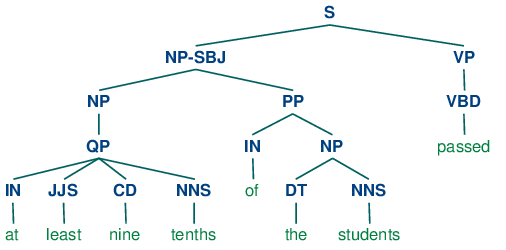b.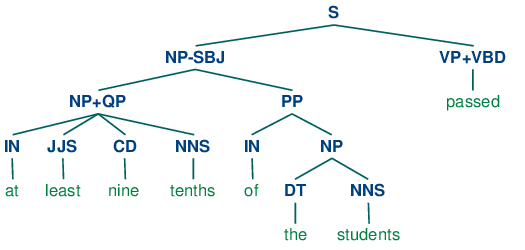c.